## ML Aggarwal Class 6 Solutions for ICSE Maths Chapter 15 Data Handling Check Your Progress

Question 1.
A die is thrown 25 times and the scores were as given below :
2, 1, 4, 6, 2, 3, 1, 5, 6, 3, 4, 5, 2, 1, 6, 6, 6, 3, 2, 2, 2, 4, 3, 2, 2
(i) Construct data array.
(ii) Construct tally chart and frequency distribution table.
Solution:
(i) Data Array is constructed by writing the scores in the ascending order.
1, 1, 1, 2, 2, 2, 2, 2, 2, 2, 2, 3, 3, 3, 3, 4, 4, 4, 5, 5, 6, 6, 6, 6, 6
(ii) Tally chart and frequency distribution table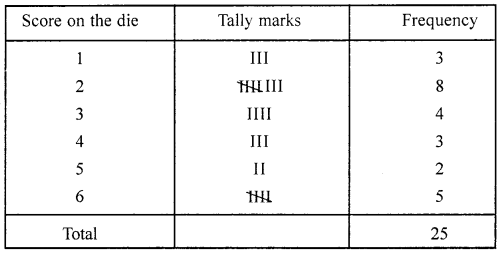Question 2.
In primary school, the number of students in different classes are as follows: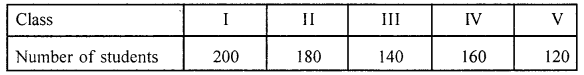Represent this data by a pictograph, using≃ 20 students.
Solution:
The pictograph for the given information is: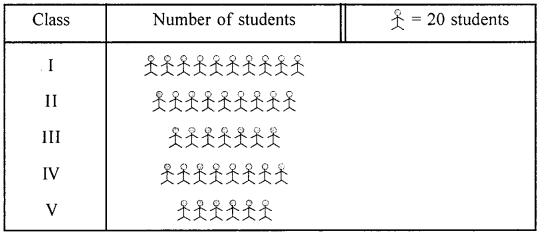Question 3.
Observe the following bar graph, showing the marks scored by Gurmeet in the annual examination in different subjects:Answer the following questions: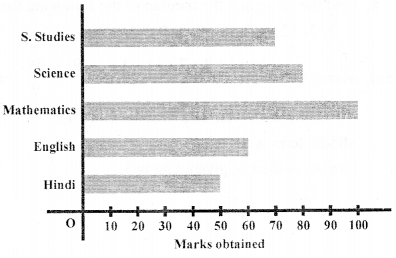(i) What is the scale of this bar graph?
(ii) In which subject Gurmeet obtained maximum marks?
(iii) In which subject she obtained minimum marks?
(iv) Name the subject(s) in which she got 80 or more marks.
Solution:
(i) Scale: 1 unit length = 10 marks obtained u io
(ii) Mathematics
(iii) Hindi
(iv) Science and Mathematics

Question 4.
The following table shows the monthly expenditure of a family on various items: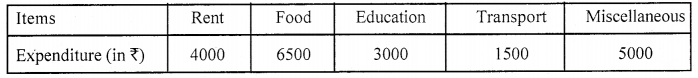Represent the data by a bar graph.
Solution: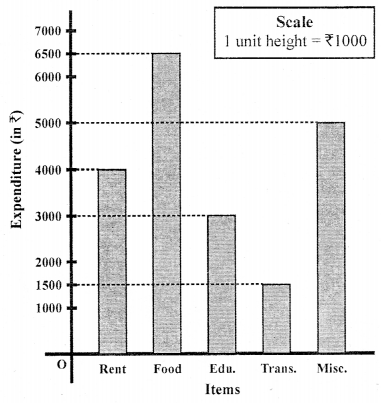Question 5.
Find the mean and the median of the following data:
5, 3, 12, 0, 7, 11, 4, 3, 9
Solution:
Mean = $$\frac{5+3+12+0+7+11+4+3+9}{9}=\frac{54}{9}$$= 6
Arranging the given data in ascending order: 0, 3, 3, 4, 5, 7, 9, 11, 12
Middle term is 5
Hence, median of given data is 5.